#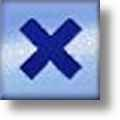Multiplication Worksheets

## Dynamically Created Multiplication Worksheets

Here is a graphic preview for all of the multiplication worksheets. You can select different variables to customize these multiplication worksheets for your needs. The multiplication worksheets are randomly created and will never repeat so you have an endless supply of quality multiplication worksheets to use in the classroom or at home. Our multiplication worksheets are free to download, easy to use, and very flexible.

## Quick Link for All Multiplication Worksheets

Click the image to be taken to that Multiplication Worksheet.

##### Multiplication Times TableCharts Worksheets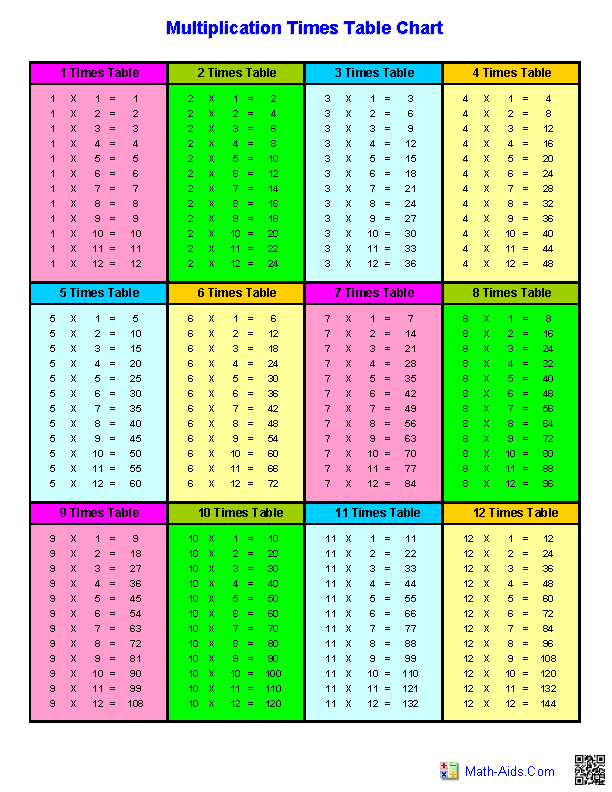##### Multiplication Times TablesSized Chart##### Multiplication Times TablesPractice Worksheets##### Multiplication Times TablesTimed Drills Worksheets##### Multiplication Times TablesAdvanced Timed Drills##### Multiplication Times TablesTarget Circles Worksheets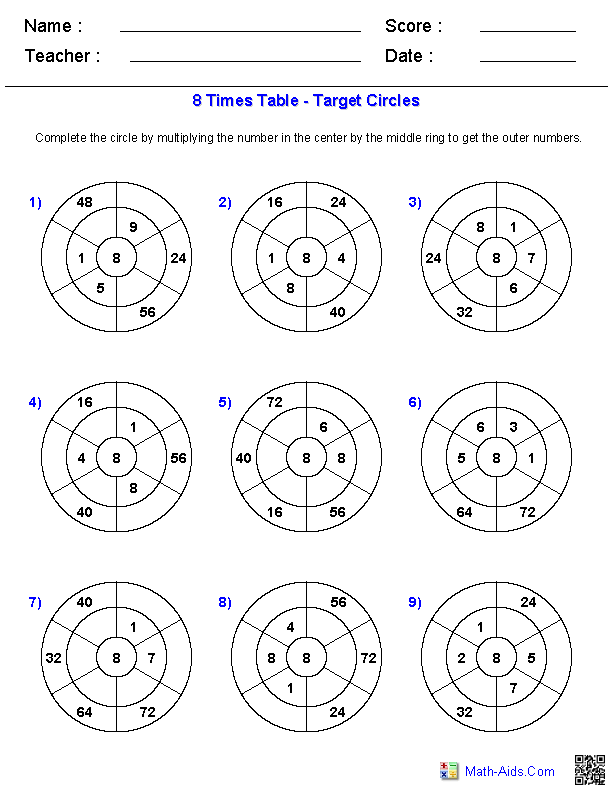##### Single DigitMultiplication Worksheets##### Multiplication Problems 0-12Multiplication Worksheets##### Multiple DigitMultiplication Worksheets##### Single or Multiple DigitMultiplication Worksheets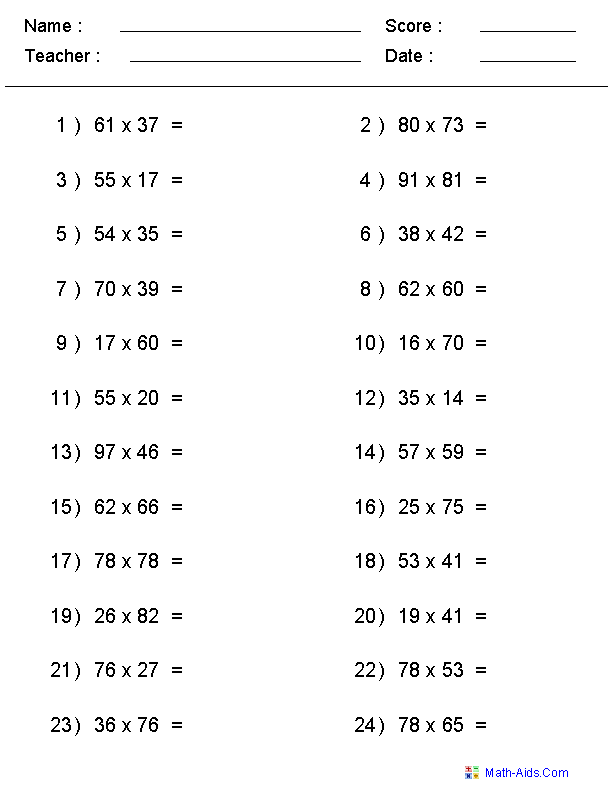##### 1, 3, or 5 Minute DrillsMultiplication Worksheets##### Missing FactorMultiplication Worksheets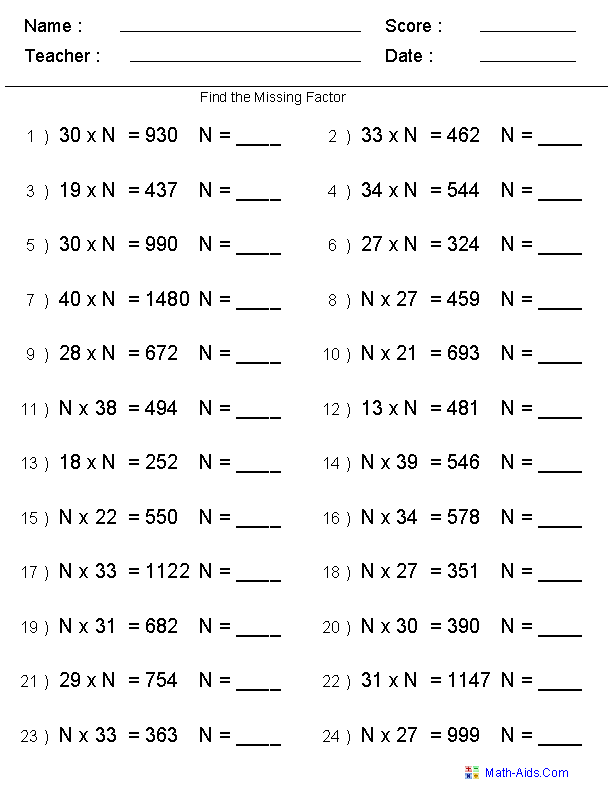##### Missing FactorDifferent Formats##### Decimal NumberMultiplication Worksheets##### Negative NumberMultiplication Worksheets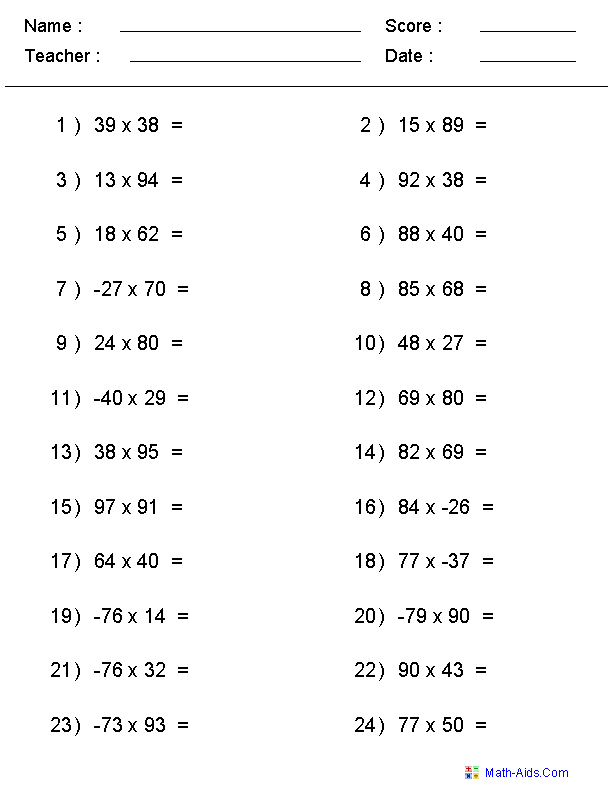##### Multiples of Ten MultiplicationMultiplication Worksheets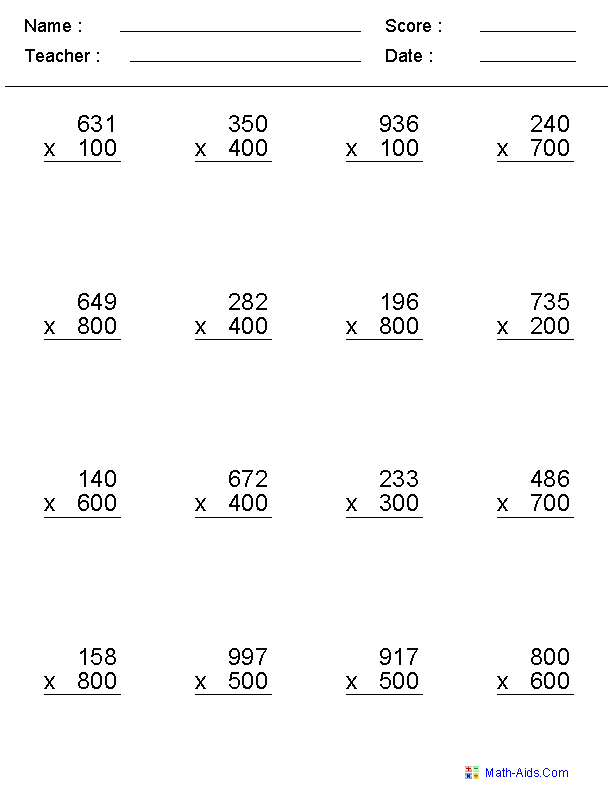##### Multiplying with Powersof Ten Worksheets##### Multiplying MoneyMultiplication Worksheets##### Learning Multiplicationwith Arrays Worksheets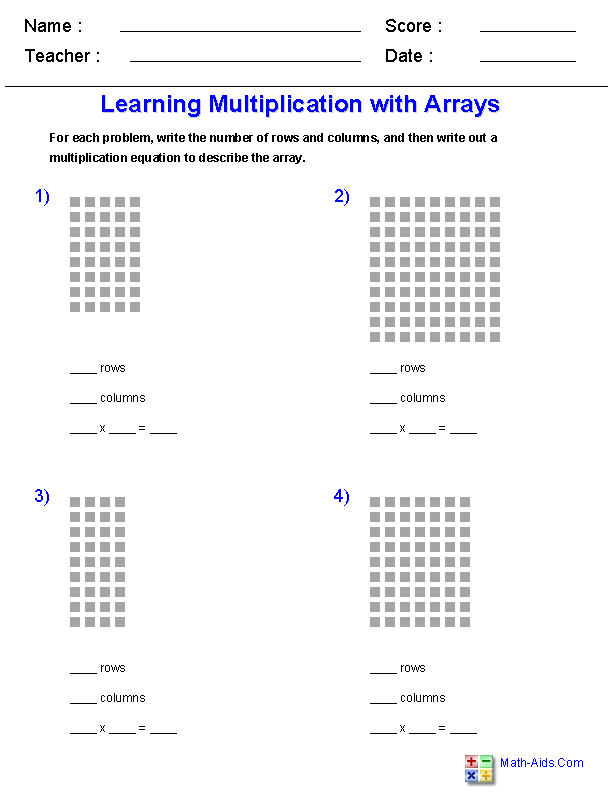##### Commutative Propertyof Multiplicationwith Arrays Worksheets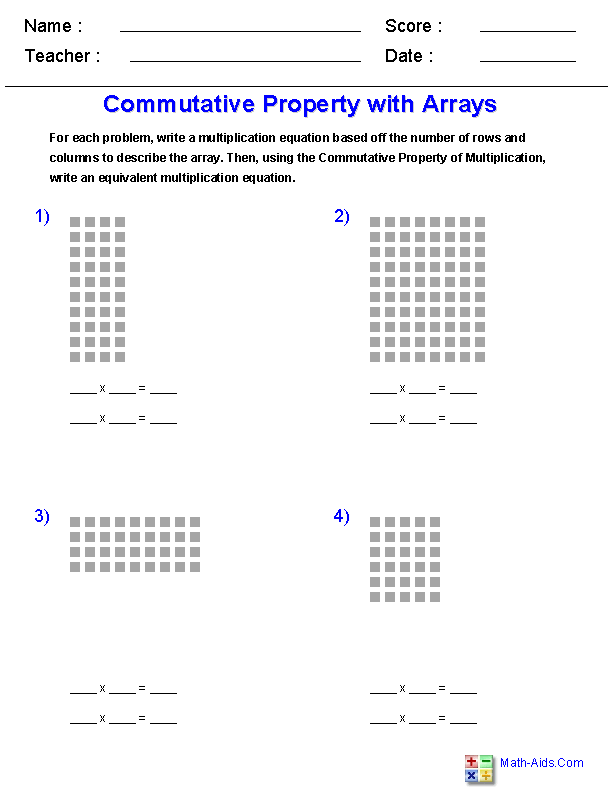##### Advanced Multiplication Factswith Arrays Worksheets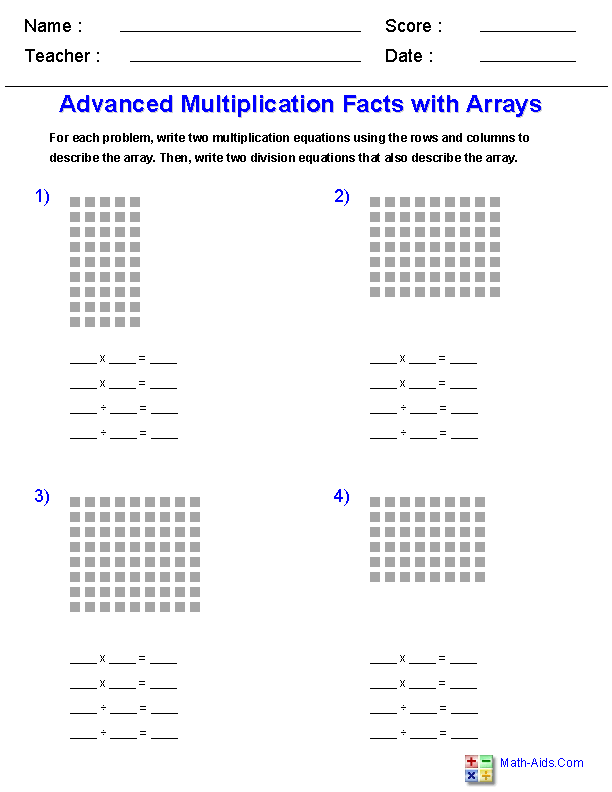##### Drawing and Determiningwith Arrays Worksheets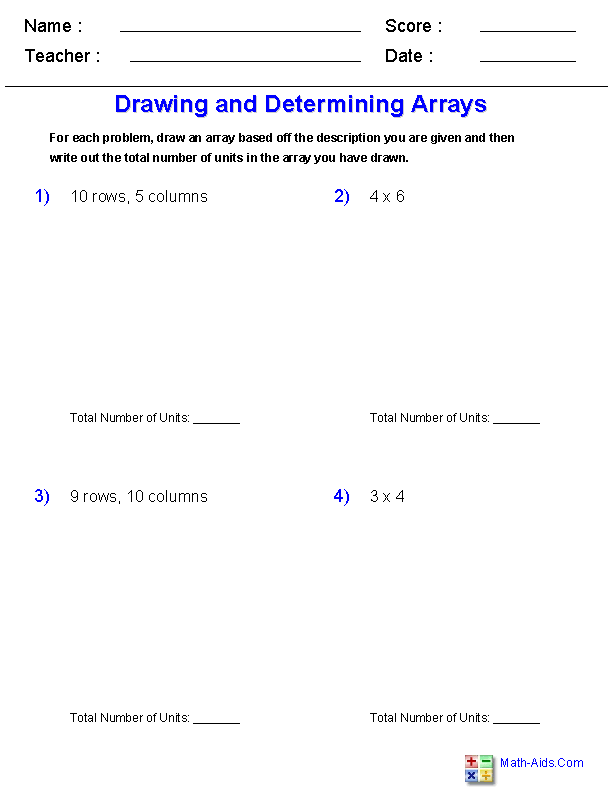##### Word Problemswith Arrays Worksheets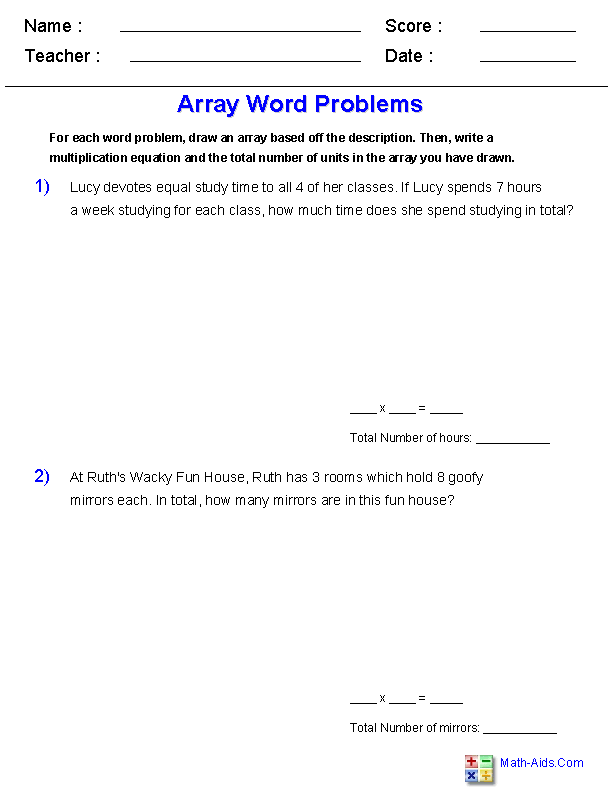##### Zero to 99 FactsMultiplication Worksheets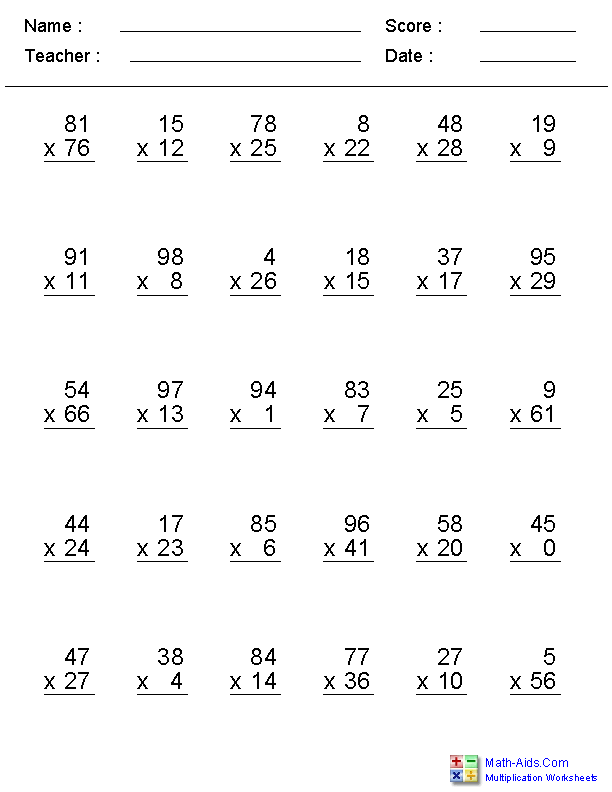Recommended Videos

## Detailed Description for All Multiplication Worksheets

Multiplication Times Table Worksheets for Lesson Plans
These multiplication times table worksheets are colorful and a great resource for teaching kids their multiplication times tables. A complete set of free printable multiplication times tables for 1 to 12. These multiplication times table worksheets are appropriate for Kindergarten, 1st Grade, 2nd Grade, 3rd Grade, 4th Grade, and 5th Grade.

Multiplication Times Tables Sized Chart
This multiplication times table charts is a great resource for teaching kids their multiplication times tables. The chart is sized based off the magnitude of the multiplied number. This multiplication chart is great for a visual representation of the multiplication times tables. This multiplication chart is appropriate for Kindergarten, 1st Grade, 2nd Grade, 3rd Grade, 4th Grade, and 5th Grade.

Multiplication Times Tables Practice Worksheets
These multiplication times table practice worksheets may be used with four different times table ranges starting at 1 through 9 and going up to 1 through 12. The numbers in the Multiplication Times Table Worksheets may be selected to be displayed in order or randomly shuffled. The multiplication worksheets times tables may be fully displayed to use as a teaching example or completely hidden for the students to use as practice. These multiplication worksheets are great to test your students understanding of the multiplication times tables. These multiplication worksheets are appropriate for Kindergarten, 1st Grade, 2nd Grade, 3rd Grade, 4th Grade, and 5th Grade.

Multiplication Times Tables Timed Drills Worksheets
These multiplication times table worksheets are for testing the students knowledge of the times tables. A student should be able to work out the 20 problems correctly in 1 minute. You may select which multiplication times table to use. These multiplication worksheets are appropriate for Kindergarten, 1st Grade, 2nd Grade, 3rd Grade, 4th Grade, and 5th Grade.

Advanced Multiplication Times Tables Drills Worksheets
These worksheets will generate multiple times tables drills as selected by the user.
The user may select from 256 different multiplication problems from times tables ranging from 0 to 15.
The user may also select a 1 minute drill of 20, 3 minute drills of 60 problems, or 5 minute drill of 100 problems, or a custom drill with ranges from 20 to 100 problems and times of 1 to 5 minutes. These multiplication worksheets are appropriate for Kindergarten, 1st Grade, 2nd Grade, 3rd Grade, 4th Grade, and 5th Grade.

Multiplication Times Tables Target Circles Worksheets
These worksheets will generate nine times tables target circles drills as selected by the user.
The user may select from times tables ranging from 1 to 15, and the range for the table to be between 0 and 12.

Single Digit Multiplication Worksheets
Vertical Format

These single digit multiplication worksheets are configured for a vertical problem format. The numbers for each factor may be individually varied to generate different sets of multiplication worksheets. These multiplication worksheets are appropriate for Kindergarten, 1st Grade, 2nd Grade, 3rd Grade, 4th Grade, and 5th Grade.

Multiplication Worksheets For Problems in the Range of 0 to 12
Vertical Format

These multiplication worksheets are configured to produce problems in the range of 0 thru 12 in a vertical format. The numbers for each factor may be individually varied to generate different sets of Multiplication problems. If you select the number 5 in the one group and all of the numbers 0 through 12 in the other group, then you will produce a multiplication worksheet that generates problems for the 5 times tables. These multiplication worksheets are appropriate for Kindergarten, 1st Grade, 2nd Grade, 3rd Grade, 4th Grade, and 5th Grade.

Multiple Digits Multiplication Worksheets
Vertical Format

These multiplication worksheets may be configured for 2, 3, or 4 digit multiplicands being multiplied by 1, 2, or 3 digit multipliers. You may select between 12 and 25 multiplication problems to be displayed on the multiplication worksheets. These multiplication worksheets are appropriate for Kindergarten, 1st Grade, 2nd Grade, 3rd Grade, 4th Grade, and 5th Grade.

Single or Multiple Digit Multiplication Worksheets
Horizontal Format

These multiplication worksheets may be configured for either single or multiple digit horizontal problems with 2 factors. You may select between 12 and 30 multiplication problems to be displayed on the multiplication worksheets. These multiplication worksheets are appropriate for Kindergarten, 1st Grade, 2nd Grade, 3rd Grade, 4th Grade, and 5th Grade.

1, 3, or 5 Minute Drill Multiplication Worksheets
Number Range (0 - 12)

A timed drill is a multiplication worksheet with all of the single digit multiplication problems on one page. A student should be able to work all of the problems on the multiplication worksheets correctly in the allowed time. These multiplication worksheets are appropriate for Kindergarten, 1st Grade, 2nd Grade, 3rd Grade, 4th Grade. and 5th Grade.

Vertical Format

This worksheet will generate advanced multiplication drills as selected by the user.
The user may select from 256 different multiplication problems from multiplication tables ranging from 0 to 15.
The user may also select a 1 minute drill of 20, a 3 minute drill of 60 problems, or a 5 minute drill of 100 problems, or a custom drill with ranges from 20 to 100 problems and times of 1 to 5 minutes.

Missing Factor Multiplication Worksheets
Horizontal Format

These multiplication worksheets are a good introduction for algebra concepts. You may select various types of characters to replace the missing factor for the multiplication worksheets. The formats of the problems are horizontal and the answers range from 0 to 99. You may vary the numbers of multiplication problems on the multiplication worksheets from 12 to 30. These multiplication worksheets are appropriate for Kindergarten, 1st Grade, 2nd Grade, 3rd Grade, 4th Grade, and 5th Grade.

Missing Factor Different Formats Multiplication Worksheets
Horizontal Format

These multiplication worksheets are great for building missing factor problems. You may select different formats for the multiplication problems and the range of numbers to use. The formats of the problems are horizontal and you may select 12, 16, 20, 24 or 30 multiplication problems per worksheet.

Decimal Number Multiplication Worksheets
Vertical Format

These multiplication worksheets may be configured for 1 or 2 Digits on the right of the decimal and up to 2 digits on the left of the decimal. You may vary the numbers of multiplication problems on the multiplication worksheets from 12 to 25. These multiplication worksheets are appropriate for Kindergarten, 1st Grade, 2nd Grade, 3rd Grade, 4th Grade, and 5th Grade.

Negative Number Multiplication Worksheets
Horizontal Format

These multiplication worksheets may be configured for either single or multiple digit horizontal problems. The factors may be selected to be positive, negative or mixed numbers for these multiplication worksheets. You may vary the numbers of multiplication problems on the multiplication worksheets from 12 to 30. These multiplication worksheets are appropriate for Kindergarten, 1st Grade, 2nd Grade, 3rd Grade, 4th Grade, and 5th Grade.

Multiples of Ten Multiplication Worksheets
Vertical Format

These multiplication worksheets may be configured for 2, 3, or 4 digit multiplicands being multiplied by multiples of ten. You may vary the numbers of multiplication problems on the multiplication worksheets from 12 to 20. These multiplication worksheets are appropriate for Kindergarten, 1st Grade, 2nd Grade, 3rd Grade, 4th Grade, and 5th Grade.

Multiplying with Powers of Ten Multiplication Worksheets
Horizontal Format

These multiplication worksheets may be configured for 2, 3, or 4 digit multiplicands being multiplied by multiples of ten that you choose from a table. You may vary the numbers of problems on the worksheet from 15 to 27. These multiplication worksheets are appropriate for Kindergarten, 1st Grade, 2nd Grade, 3rd Grade, 4th Grade, and 5th Grade.

Multiplying Money Worksheets
Vertical Format

These multiplication worksheets may be configured for up to 3 digits on the left of the decimal. The currency symbol may be selected from Dollar, Pound, Euro, and Yen. You may vary the numbers of problems for each worksheet from 12, 16 or 20. These multiplication worksheets are appropriate for Kindergarten, 1st Grade, 2nd Grade, 3rd Grade, 4th Grade, and 5th Grade.

Learning Multiplication with Arrays Worksheets
These multiplication worksheets use arrays to help teach multiplication and how to write out multiplication equations. The student will be given an array and asked to write out the numbers of rows and columns in the array, as well as a multiplication equation to describe the array. You may select the range of rows and columns used for the arrays. These multiplication worksheets are appropriate for 3rd Grade, 4th Grade, and 5th Grade.

Commutative Property of Multiplication with Arrays Worksheets
These multiplication worksheets use arrays to help teach the commutative property of multiplication and how to write out multiplication equations. The student will be given an array and asked to write out a multiplication equation and then using the commutative property of multiplication, find an equivalent multiplication equation. You may select the range of rows and columns used for the arrays. These multiplication worksheets are appropriate for 3rd Grade, 4th Grade, and 5th Grade.

Advanced Multiplication Facts with Arrays Worksheets
These multiplication worksheets use arrays to help teach how to write out multiplication and division equations. The student will be given an array and asked to write out multiplication and division equations to describe the array using the rows and columns as guidance. You may select the range of rows and columns used for the arrays. These multiplication worksheets are appropriate for 3rd Grade, 4th Grade, and 5th Grade.

Drawing and Determining Arrays Worksheets
These multiplication worksheets help teach multiplication by learning how to draw and determine the size of arrays. The student will be given a description of an array and then asked to both draw the array and determine the number of units in the array. You may select the range of rows and columns used for the arrays, as well as the description given to draw the array. These multiplication worksheets are appropriate for 3rd Grade, 4th Grade, and 5th Grade.

Array Word Problems Worksheets
These multiplication worksheets help teach multiplication by learning how to draw and determine the size of arrays based off a given word problem. The student will be given a word problem in which they must draw an array and write a multiplication equation to describe and solve the word problem. You may select the range of rows and columns used for the arrays. These multiplication worksheets are appropriate for 3rd Grade, 4th Grade, and 5th Grade.

Zero to 99 - 2 Factor Multiplication Worksheets
These Multiplication worksheets will produce 2 factor problems in a vertical format where you may select numbers from zero to 99 to be used in the problems. The numbers for each factor may be individually varied to generate different sets of Multiplication problems. You may select up to 30 Multiplication problems per worksheet. These multiplication worksheets are appropriate for 3rd Grade, 4th Grade, and 5th Grade.